The gain A of a voltage amplifier is the ratio between the output signal and the input signal. More formally, we have: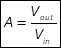Sometimes it is convenient to express the gain in dB as follows:.

The gain of a voltage amplifier depends on the specific characteristics of the vacuum tube used, and on the load resistance. Datasheets of electronic vacuum tubes generally specify some useful parameters to determine how the signal applied to the grid is amplified. Two very useful parameters are the amplification factor μ and the anode resistance (or plate resistancera.

The amplification factor is defined as the ratio between the anode voltage variation and the grid voltage variation, when the anode current is maintained constant: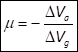, with Ia constant.

The amplification factor represents the maximum possible voltage amplification for a vacuum tube, which can be ideally (but not practically) obtained using a load with infinite resistance. This parameter is very stable on various operating conditions of the vacuum tube. The minus sign indicates that the anode voltage phase is inverted with respect to the grid voltage phase.

The other useful parameter is the anode resistance. It is defined as ratio between the anode voltage variation and the anode current variation, when the grid voltage is maintained constant:, with Vg constant.

The anode resistance is the resistance offered by the vacuum tube when the grid voltage is maintained constant and the anode voltage varies. It can be represented as a series resistance between the anode of the vacuum tube and the anode pin. Its value is not stable and depends on the specific operating conditions. Vacuum tubes datasheets, generally, report various values of the anode resistance, in correspondence of various anode voltages. If the datasheet does not report the anode resistance for the chosen configuration, it can be obtained from the anode characteristic graph, using previous equation.

When a load is connected to the anode, the real gain of the amplifier is smaller than the amplification factor μ. In fact, as long as the loadline becomes more vertical, the anode voltage variation, in correspondence of the same grid voltage variation, becomes smaller. For instance, previously we discussed that when the load resistance is zero the loadline is vertical, so no voltage amplification occurs. The real voltage gain A of the voltage amplifier, which is the ratio between the anode voltage variation and the grid voltage variation, in presence of a load, can be computed using the amplification factor μ, the anode resistance ra, and the value of the load resistance RL.

Let Vg be the grid bias voltage and Vq the corresponding quiescent anode voltage. When the AC input signal Vin is added to the grid bias voltage Vg, the anode voltage becomes Vq + Vout. The value of Vout can be determined using the equivalent circuit in Figure 9, where the vacuum tube is replaced by an AC power supply. In the equivalent circuit, the grid bias, the quiescent anode voltage, and the power supply V+ are not considered, given that they are constant. The voltage of the AC power supply (which replaces the vacuum tube) is equal to the amplification factor times the input voltage –μVin. The minus sign here indicates that the phase of the AC power supply is reversed with respect to that of Vin. The output amplified signal Vout is taken from the voltage divider formed by the anode resistance ra and the load resistance RL. Its value is obtained using the voltage divider equation as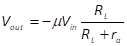.

Finally, using the equation for the voltage gain we have: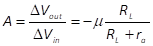.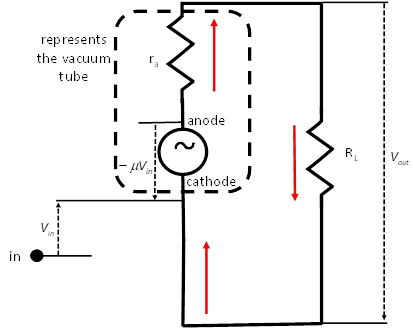Figure 9: Equivalent circuit of the voltage amplifier.The vacuum tube is seen as an AC voltage source equal to the amplification factor μ times the input signal Vin, with opposite phase. The anode resistance and the load resistance are in series to the voltage source to form a voltage divider. The output amplified signal Vout is taken at the voltage divider.

Generally, the above equation is reported without the minus sign, given that we are mainly interested in the amplitude of the signal, rather than its phase. So, we have the following equation for the voltage gain of an amplifier:Example 2: Gain of a voltage amplifier Consider for instance a 12AX7 vacuum tube and suppose it is configured according to the green loadline in Figure 8, corresponding to a load of 150K Ohm. Suppose the operating point is set with a grid bias Vg of -1.5V, corresponding to an anode voltage Va of 175V. The 12AX7 datasheet specifies an amplification factor μ=100. The anode resistance at the anode voltage of 175V, in correspondence of a grid bias of -1.5V, can be computed as follows. We take, from the anode characteristic graph, the currents in correspondence of voltages slightly lower and higher than 175V, along the plot corresponding to -1.5V grid voltage. Then, we use anode resistance equation to determine it. The current in correspondence of an anode voltage of 190V is 1.0 mA. The current in correspondence of an anode voltage of 160V is 0.6 mA. Using the anode resistance equation, we have: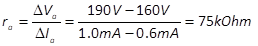. We now have all we need to estimate the gain of this voltage amplifier: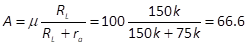.